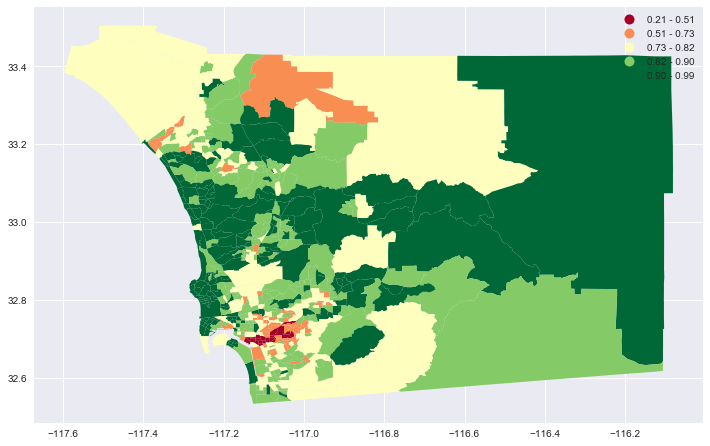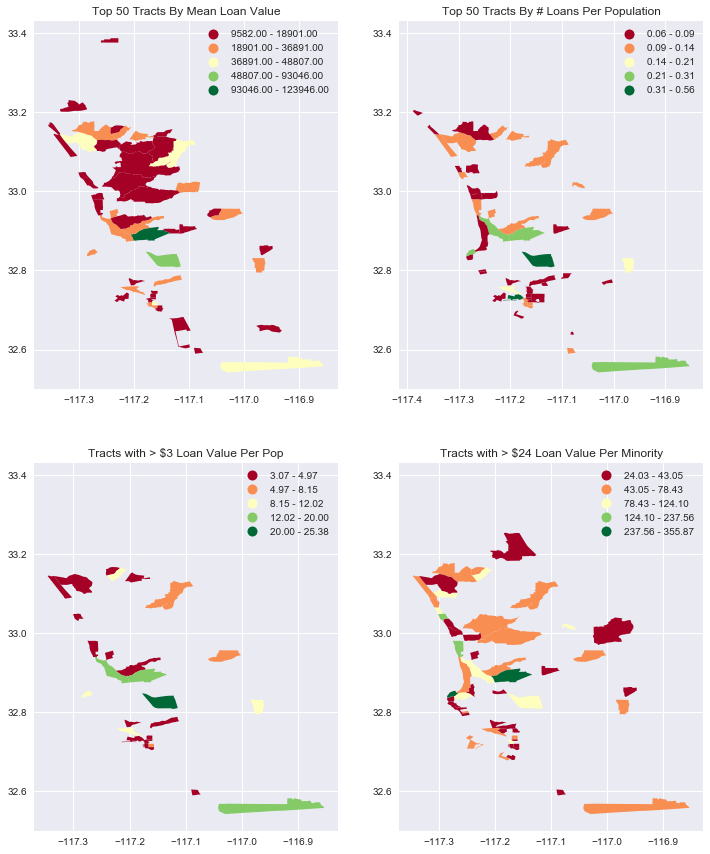# San Diego Data Knowledge Base

A demonstration of how to combine FFIEC records of business loans with census data and display the data in a variety of types of maps..

``````%load_ext metapack
%matplotlib inline

import pandas as pd
import geopandas as gpd
import numpy as np
import metapack as mp
import matplotlib.pyplot as plt
import seaborn as sns
from sdipylib.geo import *
sns.set(color_codes=True)

from pkg_resources import get_distribution
assert get_distribution('publicdata').version >= '0.1.3'``````

This notebook is a demonstration of how to combine FFIEC records of business loans with census data and display the data in a variety of type of maps. The demonstration will cover using Metatab and Metapack to access data, creating maps with GeoPandas, and creating zoomable maps with a basemap using folium.

This demonstration will focus on mapping, so if you’d like a more complete tutorial on using Metapack and Census data, please refer to the American Community Survey tutorial notebook.

First, we’ll add a Metatab configuration that defines the references to the data we’ll use, which include:

• An ACS Table for counts of people by race in tracts in San Diego county
• Tract grographic boundaries for San DIego County
• A Metapack package for CRA Business loan originations.

The American Community Survey tutorial notebook. has more information about the structure of the Metatab configuration, and the CRA Loan dataset is available in a friendly form at the Data Library’s data repository.

Note that to run this notebook, you will need to install these python packages:

``````jupyter
pandas
geopandas
matplotlib
seaborn
publicdata
``````
``````%%metatab

Dataset: cra_mapping
Origin: sandiegodata.org
Version: 1

Section: Contacts
Wrangler: Eric Busboom
Wrangler.Email: eric@sandiegodata.org
Wrangler.Organization: San Diego Regional Data Library

Section: References

Reference: censusreporter:B02001/140/05000US06073
Reference.Name: B02001
Reference.Description: Table B02001: Race, by tract, in San Diego County

Reference: censusreportergeo://B02001/140/05000US06073
Reference.Name: B02001g
Reference.Description: Table B02001: Race, by tract, in San Diego County

Reference: metapack+http://library.metatab.org/ffiec.gov-cra_aggregate_smb-orig-4.csv#sb_agg_loan_orig
Reference.Name: agg_loan_orig
Reference.Description: CRA Loan originations, aggregated to tracts.
``````

# Getting Started: Processing Data

Generally, the first parts of a Notebook should contain all of the data manipulations to get data sets combined, or or create new columns, and the the remainer of the notebook is the analysis. So, we will start by extracting data frames from the Metatab configuration. Recall from the American Community Survey tutorial notebook. that the `%metatab` cell creates the `mt_pkg` package variable, and from that package we can extract dataframes, for census data, and geoframes for geographic data.

``````# The mt_pkg var is created by the %%metatab magic.
# The B02001 and B02001g frames have the same data, but B02001 is a CensusDataFrame and B02001g is a GeoDataFrame
B02001 = mt_pkg.reference('B02001').dataframe().set_index('geoid')
B02001g = mt_pkg.reference('B02001g').geoframe().set_index('geoid')``````

Because the URL of the data frame `B02001` is special ( the `censusreporter:` scheme is defined in the `publicdata` package ) the resulting dataframe is of type `publicdata.censusreporter.dataframe.CensusDataFrame`, it has a lot of special features, including a `.title` property that expands the column names to be more sensible. This view makes it easier to figure out what columns to incorporate in later analysis.

``B02001.titles.head(2).T``
geoid 14000US06073000100 14000US06073000201
name 14000US06073000100 14000US06073000201
B02001001 Total 2716 2223
Margins for B02001001 Total 206 260
B02001002 Total White alone 2549 1983
Margins for B02001002 Total White alone 188 276
B02001003 Total Black or African American alone 0 27
Margins for B02001003 Total Black or African American alone 12 42
B02001004 Total American Indian and Alaska Native alone 0 7
Margins for B02001004 Total American Indian and Alaska Native alone 12 12
B02001005 Total Asian alone 60 95
Margins for B02001005 Total Asian alone 38 67
B02001006 Total Native Hawaiian and Other Pacific Islander alone 10 0
Margins for B02001006 Total Native Hawaiian and Other Pacific Islander alone 15 12
B02001007 Total Some other race alone 35 45
Margins for B02001007 Total Some other race alone 33 49
B02001008 Total Two or more races 62 66
Margins for B02001008 Total Two or more races 42 54
B02001009 Total Two or more races Two races including Some other race 30 9
Margins for B02001009 Total Two or more races Two races including Some other race 34 15
B02001010 Total Two or more races Two races excluding Some other race, and three or more races 32 57
Margins for B02001010 Total Two or more races Two races excluding Some other race, and three or more races 27 52

In this case, we’re going to create new variables for “Minority” and “Non Minority” where “Non Minority” is defined as White and Asian.

``````B02001['white_asian'], B02001['white_asian_m90'] = B02001.sum_m('B02001002', 'B02001005')
B02001['minority'] = B02001.B02001001 - B02001.white_asian
B02001['non_min_r'], B02001['non_min_r_m90'] = B02001.ratio('white_asian', 'B02001001')``````

For the loan data, let’s drop all of the records that are nulls, select only tract records, and select only the year 2015.

``````loans_r = mt_pkg.reference('agg_loan_orig')

loans = loans.dropna(subset=['geoid']).dropna(subset=['tract']).set_index('geoid')

# Note! Only one Year!
loans = loans[loans.year == 2015]``````
``loans_r``

### agg_loan_orig

tableidstringTable ID
yearnumberActivity Year
typenumberLoan Type
actionnumberAction Taken Type
statestringState
countystringCounty
msastringMSA/MD
tractstringCensus Tract
split_countystringSplit County Indicator
pop_classstringPopulation Classification
income_groupstringIncome Group Total
report_levelstringReport Level
number_lt100numberNumber of Small Business Loans Originated with Loan Amount at Origination < \$100,000
total_lt100numberTotal Loan Amount of Small BusinessLoans Originated with Loan Amountat Origination < \$100,000
number_100_250numberNumber of Small Business Loans Originated with Loan Amount at Origination > 100,000 and< \$250,000
total_100_250numberTotal Loan Amount of Small BusinessLoans Originated with Loan Amount at Origination > \$100,000 and < \$250,000
number_250_1mnumberNumber of Small Business Loans Originated with Loan Amount at Origination > \$250,000 and< \$1M
total_250_1mnumberTotal Loan Amount of Small BusinessLoans Originated with Loan Amount at Origination > \$250,000 and <\$1,000,000
number_bus_rev_lt1mnumberNumber of Loans Originated to SmallBusinesses with Gross AnnualRevenues < \$1 million
total_bus_rev_lt1mnumberTotal Loan Amount of Loans Originated to Small Businesses with Gross Annual Revenues < \$1 million
fillerstringFiller
geoidstringGeoid

Next, we can join the ACS, geographic, and loans dataframes. Previously, they were all given `geoid` for an index, so joining them is easy. Once they are joined, we can create some summary and derived columns. Note that the geographic dataset is joined first. This is important, because it means that the final `df` will also be a geographic dataframe.

``````df = B02001g[['geometry']].join(B02001[['non_min_r']]).join(loans)

df['number_loans'] = df.number_lt100 + df.number_100_250 + df.number_250_1m
df['total_value'] = df.total_lt100 + df.total_100_250 + df.total_250_1m
df['mean_value'] = df.total_value + df.number_loans
df['loans_per_pop'] = df.number_loans / B02001.B02001001
df['loans_per_min'] = df.number_loans / B02001.minority
df['loan_val_per_pop'] = df.total_value / B02001.B02001001
df['loan_val_per_min'] = df.total_value / B02001.minority``````
``df.head(3).T``
geoid 14000US06073000100 14000US06073000201 14000US06073000202
geometry POLYGON ((-117.194904 32.75278, -117.19471 32.... POLYGON ((-117.178867 32.75765, -117.177966 32... POLYGON ((-117.184043 32.74571, -117.183827 32...
non_min_r 0.960604 0.934773 0.932522
non_min_r_m90 0.101467 0.168156 0.129733
tableid A1-1 A1-1 A1-1
year 2015 2015 2015
type 4 4 4
action 1 1 1
state 6 6 6
county 73 73 73
msa 41740 41740 41740
tract 1 2.01 2.02
split_county N N N
pop_class L L L
income_group 13 13 12
report_level NaN NaN NaN
number_lt100 140 99 150
total_lt100 1925 1608 2162
number_100_250 2 1 4
total_100_250 300 250 490
number_250_1m 2 2 0
total_250_1m 1500 1298 0
number_bus_rev_lt1m 74 56 87
total_bus_rev_lt1m 1102 1835 1536
filler NaN NaN NaN
total_value 3725 3156 2652
number_loans 144 102 154
mean_value 3869 3258 2806
loans_per_pop 0.0530191 0.0458839 0.0328849
loans_per_min 1.34579 0.703448 0.487342
loan_val_per_pop 1.3715 1.4197 0.566304
loan_val_per_min 34.8131 21.7655 8.39241
minr_dist_deg 0.109813 0.100358 0.0965819
minr_dist_m 11276.8 10254.4 10028.5

# Creating Maps

Just as `Metapack` and the `publicdata` module worked together to turn the dataset references by the `censusreporter:` reference into a special Census data frame, the `censusreportergeo:` reference returns a Geopandas dataframe. Geopandas has a built in support for geographic operations, such as creating maps. For instance:

``````# Create the matplotlib figure and a single axis, and set the figure size
# to 12 inches wide, with the same aspect ratio as the geographic dataframe. This
# keeps the map from looking distored.
fig, ax = aspect_fig_size(df, 12)

_ = df.dropna(subset=['non_min_r'])

_.plot(ax=ax, column='non_min_r', cmap='RdYlGn',scheme='fisher_jenks', legend=True);``````
``````<matplotlib.axes._subplots.AxesSubplot at 0x113967278>
````````````from sdipylib.geo import aspect

# In this case, with multiple axes per figure, setting the aspect ratio is more manual
w = 6
a = max(aspect(_mv),aspect(_lpp))

fig = plt.figure(figsize = (2*w, 2*(w/a)))

# Doing ax2 first because it has the longer y axis, and want both plots to have the same
# y axis
_ = df.replace([np.inf, -np.inf], np.nan).dropna(subset=['loans_per_pop'])\
.sort_values('loans_per_pop', ascending=False).iloc[:50]
_.plot(ax=ax2, column='loans_per_pop', cmap='RdYlGn',scheme='fisher_jenks', legend=True)
ax2.set_title("Top 50 Tracts By # Loans Per Population")

# subplot(nrows, ncols, plot_number)
_ = df.dropna(subset=['mean_value']).sort_values('mean_value', ascending=False).iloc[:50]

_.plot(ax=ax1, column='mean_value', cmap='RdYlGn',scheme='fisher_jenks', legend=True)
ax1.set_title("Top 50 Tracts By Mean Loan Value")

_ = df.replace([np.inf, -np.inf], np.nan).dropna(subset=['loan_val_per_pop'])
_ = _[_.loan_val_per_pop>3]
_.plot(ax=ax3, column='loan_val_per_pop', cmap='RdYlGn',scheme='fisher_jenks', legend=True)
ax3.set_title("Tracts with > \$3 Loan Value Per Pop")

_ = df.replace([np.inf, -np.inf], np.nan).dropna(subset=['loan_val_per_min'])
_ = _[_.loan_val_per_min>24]
_.plot(ax=ax4, column='loan_val_per_min', cmap='RdYlGn',scheme='fisher_jenks', legend=True)
ax4.set_title("Tracts with > \$24 Loan Value Per Minority");``````Geopandas mapping is really easy, but it is hard to tell where the tracts are, because there is no base map. The folium module links Geopandas and Leaflet, and lets us put choropleth maps on a scrollable, zoomable map.

``````_ = df.replace([np.inf, -np.inf], np.nan).dropna(subset=['loan_val_per_pop'])
_ = _[_.loan_val_per_pop>3]
folium_map(_,'loan_val_per_pop', zoom_start=10, fill_color='YlGn', fill_opacity=.7)``````
``````_ = df.replace([np.inf, -np.inf], np.nan).dropna(subset=['loans_per_pop'])
_ = _.sort_values('loans_per_pop', ascending=False).iloc[:100] # don't exceed notebooks IOPub data rate
#_ = _[_.loan_val_per_pop>3]
folium_map(_,'loans_per_pop', zoom_start=10, fill_color='YlGn', fill_opacity=.7)``````